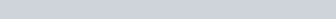ChemTalk

# Thermodynamic Processes: Types and Equations## Core Concepts

This article will cover the four thermodynamic processes: Adiabatic, Isochoric, Isothermal, and Isobaric processes along with their Pressure-Volume curves. After this article, you will be able to describe and understand how a system is able to interact with its surrounding through work and heat.

## Systems and Surroundings

In thermodynamics, a system is the entity under study. Everything else is, therefore, the surrounding of this system. A system and its surrounding can interact by exchanging matter and energy. There are three types of systems:

• Isolated Systems: are systems that are unable to exchange neither matter nor energy with their surroundings. One example of this is the thermos flask. Because the flask is sealed, no matter is exchanged. The thermos flask also prevents any form of heat transmission by preventing heat convection, conduction, and radiation. This allows the flask to keep the liquid inside of it hot for an extended period of time.
• Closed Systems: are systems that can only exchange energy with their surroundings. For example, heating pads and ice packs are closed systems. They allow for exchange of energy, which is how heat is transferred from and to them, respectively. However, the liquids inside of them do not leak out, hence, no matter is exchanged.
• Open Systems: are systems that can exchange both energy and matter with their surroundings. Such is the case of a boiling pot with no lid atop it. Energy is exchanged since the area surrounding the pot gets warmer. Moreover, some of the water vaporizes and leaves the pot, this is matter exchange.

## Notions to be Familiar with

Thermodynamic processes characterize the transfer of energy (as heat) between and within systems. They help us understand the properties of the system that change or remain constant throughout the process. But before going into the processes, we must be familiar with two things:

• The First law of thermodynamics: which states that energy is always conserved, even as changes from one form to another. This is illustrated in the equation :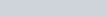where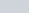is the variation of the internal energy of the system,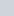is the heat added to the system, and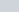is the work done by the system.
• Pressure-Volume curves: when a gas expands or is compressed, work is done by or on the system, respectively. We represent this by the equation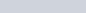Graphically, if we plot pressure (y-axis) with respect to changes in volume (x-axis), the area under the resulting curve would be equal to the value of work.

## Thermodynamic Processes

We have four processes: Isobaric, Isochoric, Isothermal, and Adiabatic

### Isobaric Process

An isobaric process is a process that occurs when pressure is constant. Since the pressure is constant irrespective of the changes in volume, then the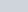curve is a horizontal flat line, and the work is the rectangular area beneath it.

### Isochoric Process (Isovolumetric)

Isochoric processes occur under constant volume. Since the volume is fixed,, ourcurve would be a vertical line with no area under it, meaning no work was done. If we placein our work equation, we would also get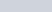.
Substituting this in the equation of the first law of thermodynamics yields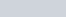. This means that the change in the energy of the system is equal to the heat that has been added to it.

### Isothermal Process

In an isothermal process, the temperature is constant. Thus, there is no change in the internal energy of the system,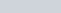. So The first law of thermodynamics simplifies to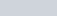, so any addition of heat to the system is compensated for by the system doing work. Thecurve of this process is a hyperbola. And one can calculate pressure from the ideal gas equation.

Adiabatic processes occur when the system and its surroundings are unable to exchange heat. Therefore,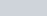. Substituting this in the first law, we get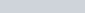. This means that the change in internal energy is equal to the work done on the system.
Thecurve -like that of isothermal processes- is also a hyperbola, but with a steeper slope.

## Practice Problems

Using the graph below, solve the following problems :

Problem 1
Identify the thermodynamic process depicted in the graph above

Problem 2
Calculate the work done using the information in the graph

## Practice Problems Solutions

Problem 1
As we can see in the graph, the pressure stays constant throughout the process. Therefore, it is an isobaric process.

Problem 2
Incurves, the area under the curve is equal to the value of work. Since this is an isobaric process, we can easy calculate the work since it takes on the shape of a rectangle. The length is the change in volume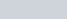, and the width is the pressure.